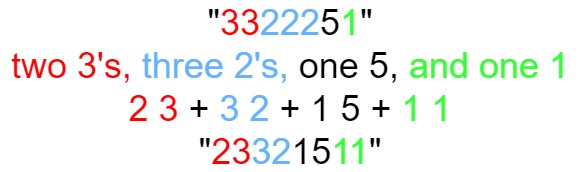## Algorithm

Problem Name: 38. Count and Say

The count-and-say sequence is a sequence of digit strings defined by the recursive formula:

• `countAndSay(1) = "1"`
• `countAndSay(n)` is the way you would "say" the digit string from `countAndSay(n-1)`, which is then converted into a different digit string.

To determine how you "say" a digit string, split it into the minimal number of substrings such that each substring contains exactly one unique digit. Then for each substring, say the number of digits, then say the digit. Finally, concatenate every said digit.

For example, the saying and conversion for digit string `"3322251"`:Given a positive integer `n`, return the `nth` term of the count-and-say sequence.

Example 1:

```Input: n = 1
Output: "1"
Explanation: This is the base case.
```

Example 2:

```Input: n = 4
Output: "1211"
Explanation:
countAndSay(1) = "1"
countAndSay(2) = say "1" = one 1 = "11"
countAndSay(3) = say "11" = two 1's = "21"
countAndSay(4) = say "21" = one 2 + one 1 = "12" + "11" = "1211"
```

Constraints:

• `1 <= n <= 30`

## Code Examples

### #1 Code Example with C Programming

```Code - C Programming```

``````
char* countAndSay(int n) {
char *s, *d, *x;
int i, c;

if (!n) return NULL;

#define BUF_SIZE 100 * 1024

s = malloc(BUF_SIZE);
x = d = malloc(BUF_SIZE);
s = '1';
s = 0;

while (n > 1) {
c = 1;
for (i = 0; s[i]; i ++) {
if (s[i] != s[i+1]) {
sprintf(x, "%d%c", c, s[i]);
x += strlen(x);
c = 1;
} else {
c ++;
}
}
x = s;
s = d;
d = x;
n --;
}

free(d);
return s;
}
``````
Copy The Code &

Input

cmd
n = 1

Output

cmd
"1"

### #2 Code Example with C++ Programming

```Code - C++ Programming```

``````
class Solution {
public:
string countAndSay(int n) {
if(n == 1) return "1";
string s = countAndSay(n - 1);
string res = "";
for(int i = 0; i < s.size(); i++){
int count = 1;
while(i < s.size() - 1 && s[i] == s[i + 1]) i++, count++;
res.append(to_string(count) + s[i]);
}
return res;
}
};

``````
Copy The Code &

Input

cmd
n = 4

Output

cmd
"1211"

### #3 Code Example with Java Programming

```Code - Java Programming```

``````
class Solution {
public String countAndSay(int n) {
String s = "1";
for (int i = 1; i < n; i++) {
StringBuilder sb = new StringBuilder();
for (int j = 1, count = 1; j <= s.length(); j++) {
if (j == s.length() || s.charAt(j - 1) != s.charAt(j)) {
sb.append(count);
sb.append(s.charAt(j - 1));
count = 1;
} else {
count++;
}
}
s = sb.toString();
}
return s;
}
}
``````
Copy The Code &

Input

cmd
n = 1

Output

cmd
"1"

### #4 Code Example with Javascript Programming

```Code - Javascript Programming```

``````
const countAndSay = function(n) {
let str = '1'
for (let i = 2; i <= n; i++) {
let tempStr = ''
let count = 0
for (let j = 0, m = str.length; j < m; j++) {
const char = str.charAt(j)
count += 1
if (char !== str.charAt(j + 1)) {
tempStr += count + char
count = 0
}
}
str = tempStr
}
return str
}
``````
Copy The Code &

Input

cmd
n = 4

Output

cmd
"1211"

### #5 Code Example with Python Programming

```Code - Python Programming```

``````
class Solution:
def countAndSay(self, n):
curr = "1"
for i in range(n - 1):
tmp, cnt = "", 1
for j, c in enumerate(curr):
if j > 0 and curr[j - 1] == c:
cnt += 1
elif j > 0:
tmp += str(cnt) + curr[j - 1]
cnt = 1
if j == len(curr) - 1:
tmp += str(cnt) + curr[j]
curr = tmp
return curr
``````
Copy The Code &

Input

cmd
n = 1

Output

cmd
"1"

### #6 Code Example with C# Programming

```Code - C# Programming```

``````
using System.Text;

namespace LeetCode
{
public class _038_CountAndSay
{
public string CountAndSay(int n)
{
var result = "1";
char currentCh;
int i, j, startNum;
var builder = new StringBuilder();

for (i = 1; i < n; i++)
{
currentCh = result;
startNum = 0;
for (j = 1; j < result.Length; j++)
{
if (currentCh != result[j])
{
builder.Append(j - startNum);
builder.Append(currentCh);
currentCh = result[j];
startNum = j;
}
}
builder.Append(j - startNum);
builder.Append(currentCh);

result = builder.ToString();
builder.Clear();
}

return result;
}
}
}
``````
Copy The Code &

Input

cmd
n = 4

Output

cmd
"1211"